# Olympiad Test: Visualising Solid Shapes - 2

## 10 Questions MCQ Test Mathematical Olympiad Class 8 | Olympiad Test: Visualising Solid Shapes - 2

Description
Attempt Olympiad Test: Visualising Solid Shapes - 2 | 10 questions in 20 minutes | Mock test for Class 8 preparation | Free important questions MCQ to study Mathematical Olympiad Class 8 for Class 8 Exam | Download free PDF with solutions
QUESTION: 1

### A solid shape is generated by extruding a rectangle, out of its plane. The shape will be :

Solution:

When we extrude a rectangle, a cuboid will be generated.

QUESTION: 2

### The top view will contain :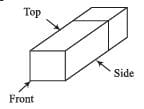Solution:

The top view will have 1 rectangle joined with a square.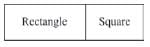QUESTION: 3

###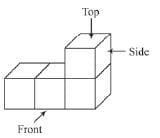The above figure is generated from 4 cubes; The number of squares in front view = x, number of squares in top view = y. (x + y) =

Solution:

Number of squares in front view = 3
Number of squares in top view = 3
∴ x + y = (3 + 3) = 6

QUESTION: 4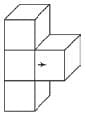A cube is extracted from a well-arranged vertical cubes, which are 3 in number. When viewed form top, the number of edges will be :

Solution:

Top view :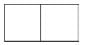Number of edges = 7

QUESTION: 5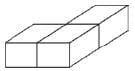The above solid contain 3 cubes. The number of edges will be : (cubes are of different dimensions)

Solution:

When 2 cubes are joined, then the number of edges will be
= (12 + 12) – (4) = 20
When 1st cube is joined to the two cubes in desired manner then no. of edges
= (20 + 12) – (4) = 28
∵ cubes are of same dimensions.

QUESTION: 6Number of vertices in the given fig. is (cubes are of same dimension)

Solution:

After joining of 2 cubes, a cuboid will be formed,
∴ No. of vertices = 8 After final joining, no. of vertices
= 8 + 4 = 12

QUESTION: 7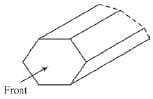The front view of the shape will have ---- edges.

Solution: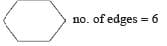QUESTION: 8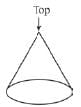The top view of a cone will be :

Solution: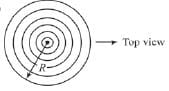The top view of a cone contains circles with radii varying from zero to R, in radial directions (same centre)

QUESTION: 9

A parallelogram is extruded outwards with the axis inclined at some angle with the vertical. The resulting figure will be :

Solution: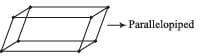QUESTION: 10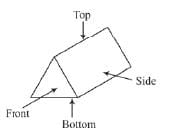The number of vertices in front view + number of vertices in top view - number of vertices in bottom view =

Solution: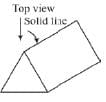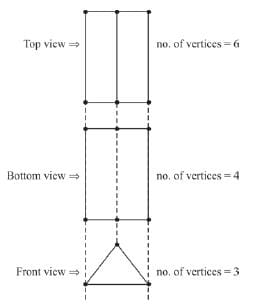∴ Required no. of vertices = (3 + 6) – (4) = 5.
∵Top view has solid line, but the solid line will be invisible in bottom view.
∴Top view has 6 vertices and bottom view has 4 vertices.Use Code STAYHOME200 and get INR 200 additional OFF Use Coupon Code